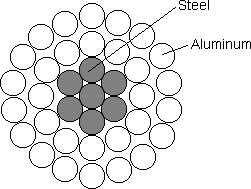QuickField

A new approach to field modelling
 Language: Global English Deutch Espanol Francais Italiano Danmark Ceske Chinese Pycckuü

>> >>

# Phase to phase fault

Phase to phase fault is one of the most widespread damages of the transmission lines in electrical networks (15-20 % from total number of failures). This model shows how to simulate the phase to phase fault in QuickField.

Problem Type:
Plane-parallel problem of transient magnetics.

Geometry:Tangent tower design. All dimensions are in meters.Wire designLine-to-line short circuit

Given:
Rated voltage (RMS) Urated = 110 kV;
Rated current (RMS) Irated = 440 A;
Line length l = 20 km;

Problem:
Determine the transient process currents after a short circuit occurred. Find the electromagnetic forces acting on the lines during this process.

Solution:
To solve this problem it is necessary to simulate both field part and circuit part. The scheme of connection of power supply phase windings, loading, elements of the circuit and short-circuit bridge is presented in the circuit model, created by the QuickField Circuit Editor.Short-circuit bridge is a QuickField block with the resistance which varies in time. This dependence is implemented using the electrical conductivity as a function of the temperature, which is also function of time - as σ(T(t)) function.Results:
Short-circuit current (amplitude) Imax = 7000 A.
Electromagnetic force of interaction between phases A and B FA-B = 1780 N/km.

Illustration of the short-circuit currents:• Video:
•Download simulation files (files may be viewed using any QuickField Edition).